# Fresnel's Equations

Fresnel's equations describe the reflection and transmission of electromagnetic waves at an interface. That is, they give the reflection and transmission coefficients for waves parallel and perpendicular to the plane of incidence. For a dielectric medium where Snell's Law can be used to relate the incident and transmitted angles, Fresnel's Equations can be stated in terms of the angles of incidence and transmission.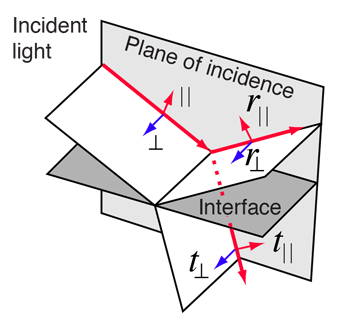For light from a medium of index n1 =
 incident upon a medium of index n2 =
 at an angle θi = °
 the angle of transmission is θt = °

Fresnel's equations give the reflection coefficients: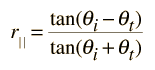= and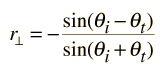=

The transmission coefficients are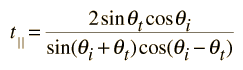= and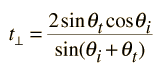=

Note that these coefficients are fractional amplitudes, and must be squared to get fractional intensities for reflection and transmission. The signs of the coefficients depend on the original choices of field directions.

You can choose values of parameters which will give transmission coefficients greater than 1, and that would appear to violate conservation of energy. (For example, try light incident from a medium of n1=1.5 upon a medium of n2=1.0 with an angle of incidence of 30°.) But the square of the transmission coefficient gives the transmitted energy flux per unit area (intensity), and the area of the transmitted beam is smaller in the refracted beam than in the incident beam if the index of refraction is less than that of the incident medium. When you take the intensity times the area for both the reflected and refracted beams, the total energy flux must equal that in the incident beam. For further details, see Jenkins and White.

Checking out conservation of energy in this situation leads to the relationshipwhich applies to both the parallel and perpendicular cases.

Parallel case: Reflected % and transmitted %.

Perpendicular case: Reflected % and transmitted %.

 Typical curves for: External reflection Internal reflection
Index

Reflection concepts

Incident light behavior

References
Hecht
Ch 4

Jenkins and White
Sec 25.2

 HyperPhysics***** Light and Vision R Nave
Go Back

# Reflection and Transmission

Typical reflection and transmission curves for external reflection. These curves are the graphical representation of the Fresnel equations. Note that the reflected amplitude for the light polarized parallel to the incident plane is zero for a specific angle called the Brewster angle. The reflected light is then linearly polarized in a plane perpendicular to the incident plane. This polarization by reflection is exploited in numerous optical devices.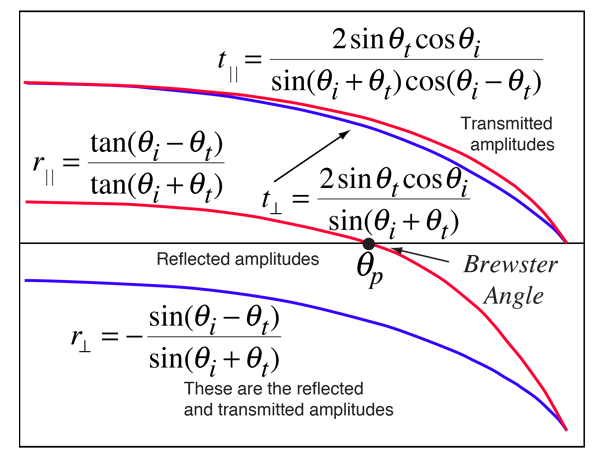Calculation Show internal reflection
Index

Reflection concepts

Incident light behavior

 HyperPhysics***** Light and Vision R Nave
Go Back

# Internal Reflection Curves

The illustration shows typical reflection curves for internal reflection. Internal reflection implies that the reflection is from an interface to a medium of lesser index of refraction, as from water to air. These curves are the graphical representation of the Fresnel equations. Note that the reflected amplitude for the light polarized parallel to the incident plane is zero for a specific angle called the Brewster angle. The reflected light is then linearly polarized in a plane perpendicular to the incident plane. This polarization by reflection is exploited in numerous optical devices. Another characteristic of internal reflection is that there is always an angle of incidence qc above which all light is reflected back into the medium. This phenomenon of total internal reflection has many practical applications in optics.Calculation Show external reflection
Index

Reflection concepts

Incident light behavior

 HyperPhysics***** Light and Vision R Nave
Go Back